# Graph Match

## Match the graphs with their equations. A self-marking, drag-and-drop mathematical exercise.

##### GradientLevel 1Level 2Level 3Exam-StyleDescriptionHelpMore Graph Activities

This is Level 3 (Mixed polynomials). Match the graphs with the corresponding equations.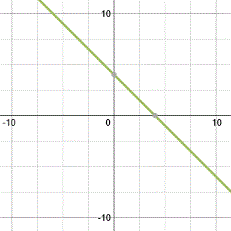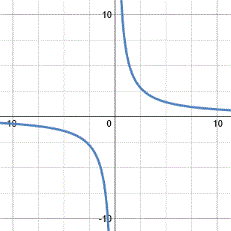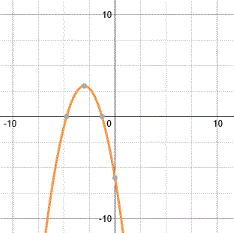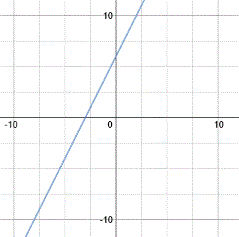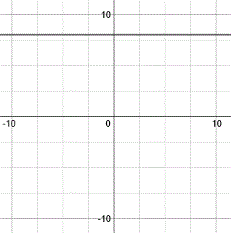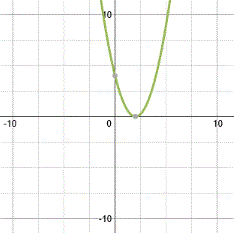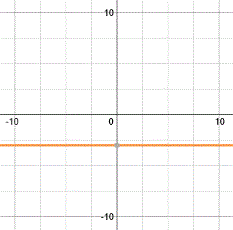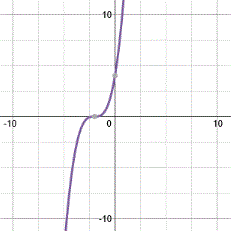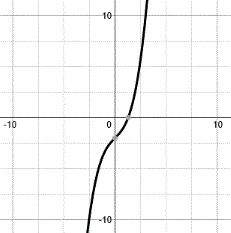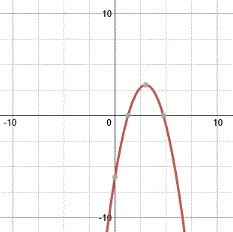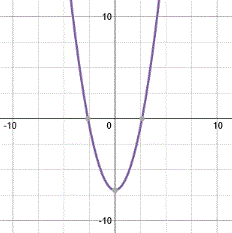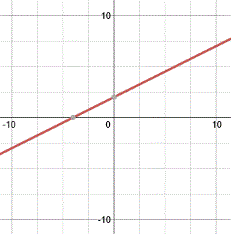$$y = 4-x$$

$$y = \frac7x$$

$$y = 3-(x+3)^2$$

$$y = 2x+6$$

$$y = 8$$

$$y = (x-2)^2$$

$$y = -3$$

$$y = \frac12 (x+2)^3$$

$$y = x+ \frac13 x^3-2$$

$$y = 3-(x-3)^2$$

$$y = x^2-7$$

$$y = \frac12 x + 2$$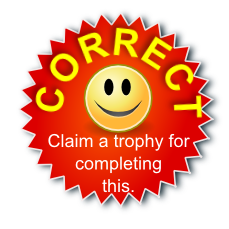The diagrams were created using the online Graph Plotter.

This is Graph Match level 3. You can also try:
Gradient Level 1 Level 2 Exam-style Questions

## Description of Levels

CloseGradient - A pre-requisite for doing the graph exercises is being able to calculate the gradient of a line.

Level 1 - Linear graphs and equationsLevel 2 - Linear and quadratic graphs and equationsLevel 3 - Mixed polynomialsExam Style questions are in the style of GCSE or IB/A-level exam paper questions and worked solutions are available for Transum subscribers.

More on this topic including lesson Starters, visual aids and investigations.

Answers to this exercise are available lower down this page when you are logged in to your Transum account. If you don’t yet have a Transum subscription one can be very quickly set up if you are a teacher, tutor or parent.

For straight line graphs arrange the equation in the form $$y = mx + c$$ where $$m$$ represents the gradient of the line and $$c$$ the y-intercept.

Maybe this video will remind you of some of the techniques for recognising graphs.

This video is from the ukmathsteacher YouTube channel.

The most important thing is to talk to your teacher if there is anything you don't understand about this topic.

Answers to this exercise are available lower down this page when you are logged in to your Transum account. If you don’t yet have a Transum subscription one can be very quickly set up if you are a teacher, tutor or parent.

Close## Transum.org

This web site contains over a thousand free mathematical activities for teachers and pupils. Click here to go to the main page which links to all of the resources available.

Please contact me if you have any suggestions or questions.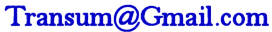## More Activities:

Mathematicians are not the people who find Maths easy; they are the people who enjoy how mystifying, puzzling and hard it is. Are you a mathematician?

Comment recorded on the 1 August 'Starter of the Day' page by Peter Wright, St Joseph's College:

"Love using the Starter of the Day activities to get the students into Maths mode at the beginning of a lesson. Lots of interesting discussions and questions have arisen out of the activities.
Thanks for such a great resource!"

Comment recorded on the 10 September 'Starter of the Day' page by Carol, Sheffield PArk Academy:

"3 NQTs in the department, I'm new subject leader in this new academy - Starters R Great!! Lovely resource for stimulating learning and getting eveyone off to a good start. Thank you!!"

#### Strategy GamesWe have collected together lots of strategy games for you to enjoy. Many of them have mathematical principles involved in their design so would make a good activity for a Mathematics lesson.

There are answers to this exercise but they are available in this space to teachers, tutors and parents who have logged in to their Transum subscription on this computer.

A Transum subscription unlocks the answers to the online exercises, quizzes and puzzles. It also provides the teacher with access to quality external links on each of the Transum Topic pages and the facility to add to the collection themselves.

Subscribers can manage class lists, lesson plans and assessment data in the Class Admin application and have access to reports of the Transum Trophies earned by class members.

If you would like to enjoy ad-free access to the thousands of Transum resources, receive our monthly newsletter, unlock the printable worksheets and see our Maths Lesson Finishers then sign up for a subscription now:

Subscribe

## Go Maths

Learning and understanding Mathematics, at every level, requires learner engagement. Mathematics is not a spectator sport. Sometimes traditional teaching fails to actively involve students. One way to address the problem is through the use of interactive activities and this web site provides many of those. The Go Maths main page links to more activities designed for students in upper Secondary/High school.

## Teachers

If you found this activity useful don't forget to record it in your scheme of work or learning management system. The short URL, ready to be copied and pasted, is as follows:

Do you have any comments? It is always useful to receive feedback and helps make this free resource even more useful for those learning Mathematics anywhere in the world. Click here to enter your comments.For All: